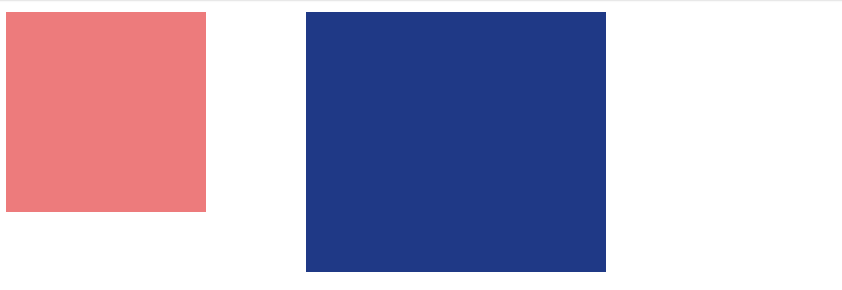#2019 iT 邦幫忙鐵人賽DAY 27
1
Modern Web

# SVG-transform

svg-transform和css-transform有異曲同工之妙，有`translate/位移``scale/縮放``rotate/旋轉``skew/傾斜`屬性

## translate/位移

translate(tx,ty)，tx和ty可以用“，”隔開，也可以直接空白，tx=x軸位移距離，ty=y軸位移距離，ty為選擇性參數，可寫可不寫，如果沒寫，預設值為0，單位為目前用戶坐標系單位。

``````<svg>
<rect fill="#EF7A7A" width="100" height="100" x="0" y="0" />
<rect fill="#1E3788" width="100" height="100" x="0" y="0" transform="translate(100,50)" />
</svg>
``````## scale/縮放

scale(sx,sy)，sx和sy可以用“，”隔開，也可以直接空白，如果只寫一個值，即長寬都以同一倍率縮放，sx為X軸縮放倍率，sy為Y軸縮放倍率，單位為目前用戶坐標系單位。[注意：使用scale時，座標也會被縮放，位置可能會有位移]

``````<svg width="500" height="500">
<rect fill="#EF7A7A" width="100" height="100" x="0" y="0" />
<rect fill="#1E3788" x="100" y="0"  width="100" height="100" transform="scale(1.5,1.3)" />
</svg>
``````## rotate/旋轉

rotate(angle,[cx,cy])，angle為旋轉角度，這裡不需要寫角度，預設就為角度，(cx,cy)為選轉圓心角度，預設為當前用戶坐標的原點，通常為SVG畫布左上角座標(0,0)，一樣不需要寫單位。

``````<svg width="500" height="500">
<rect fill="#EF7A7A" width="100" height="100" x="0" y="0" />
<rect fill="#1E3788" x="100" y="0"  width="100" height="100" transform="rotate(30)" />
</svg>
``````## skew/傾斜

``````<svg width="500" height="500">
<rect fill="#EF7A7A" width="100" height="100" x="0" y="0" />
<rect fill="#1E3788" x="100" y="0"  width="100" height="100" transform="skewX(30)" />
</svg>
``````～SVG龜速學習中，如有疑問或是錯誤，歡迎不吝指教～